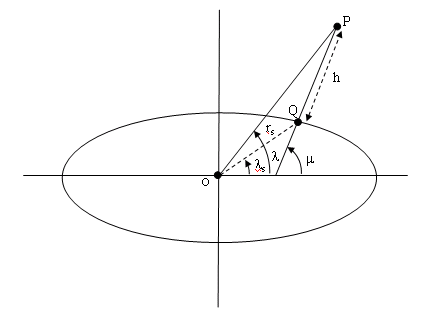Estimate radius of ellipsoid planet at geocentric latitude

• Library:
• Aerospace Blockset / Flight Parameters

•Description

The Radius at Geocentric Latitude block estimates the radius $\left({r}_{s}\right)$ of an ellipsoid planet at a particular geocentric latitude $\left({\lambda }_{s}\right)$.The following equation estimates the ellipsoid radius $\left({r}_{s}\right)$ using flattening $\left(\overline{f}\right)$, geocentric latitude $\left({\overline{\lambda }}_{s}\right)$, and equatorial radius $\left(\overline{R}\right)$:

${r}_{s}=\sqrt{\frac{{R}^{2}}{1+\left[1/{\left(1-f\right)}^{2}-1\right]{\mathrm{sin}}^{2}{\lambda }_{s}}}.$

Ports

Input

expand all

Geocentric latitude, specified as a scalar or vector, in degrees.

Data Types: double

Output

expand all

Radius of planet at geocentric latitude, returned as a scalar or vector, in the same units as flattening.

Data Types: double

Parameters

expand all

Output units, specified as:

Metric (MKS) MetersMeters
English FeetFeet

Dependencies

To enable this parameter, set Planet model to Earth (WGS84).

Programmatic Use

 Block Parameter: units Type: character vector Values: 'Metric (MKS)' | 'English' Default: 'Metric (MKS)'

Planet model to use, Custom or Earth (WGS84).

Programmatic Use

 Block Parameter: ptype Type: character vector Values: 'Earth (WGS84)' | 'Custom' Default: 'Earth (WGS84)'

Flattening of the planet, specified as a double scalar.

Dependencies

To enable this parameter, set Planet model to Custom.

Programmatic Use

 Block Parameter: F Type: character vector Values: double scalar Default: 1/298.257223563

Radius of the planet at its equator, in the same units as the desired units for ECEF position.

Dependencies

To enable this parameter, set Planet model to Custom.

Programmatic Use

 Block Parameter: R Type: character vector Values: double scalar Default: 6378137

 Stevens, Brian L., Frank L. Lewis. Aircraft Control and Simulation, New York, John Wiley & Sons, 1992.

 Zipfel, Peter H., Modeling and Simulation of Aerospace Vehicle Dynamics. Second Edition. Reston, VA: AIAA Education Series, 2000.

Aerospace Blockset DocumentationGet trial now